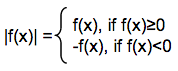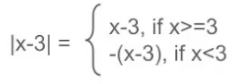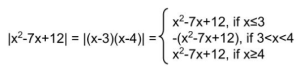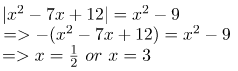# Solving Absolute Value Equations

[latexpage]
In this post we will see how to solve problems on absolute value(modulus) equations and inequalities. To understand the basics of absolute value click here – Absolute Value

#### Solving absolute value problems of the type |f(x)| + g(x) = k

How to find the value of x in absolute equations involving |f(x)| + g(x) = k

We can approach these kinds of problems by making the equation independent of modulus sign.
To make the equation independent of the absolute value sign, lets first have a look at the definition of Absolute value of f(x)We know that absolute value is always postive,
So if f(x)≥0, the value of f(x) is postive and hence |f(x)| will be equal to f(x), and if f(x)<0, the value of is negative, therefore |f(x)| will be equal to -f(x), to make the resulting value positive.

Hence, based on the definition of absolute value of f(x) we can identify those values of x, when f(x) changes its sign and based on which we can categorize our to approach to the problem into cases,
 Case 1 : f(x) ≥ 0 When f(x)≥0, |f(x)| = f(x). Hence,

|f(x)| + g(x) = k => f(x) + g(x) = k.

Case 2 : f(x) < 0 When f(x)≥0, |f(x)| = f(x). Hence, |f(x)| + g(x) = k => -f(x) + g(x) = k.

Now we have two equations in f(x) which is independent of the modulus sign. We can now solve these equations for x.

Note : Once you solve for the value of x under each case, verify if x satisfies the condition for that case. If x satisfies the condition, then it is a valid solution.

1. Solve for x: |x-3| + 2x = 7
We have,Hence,
Case 1 : x ≥ 3
|x-3| + 2x = 7 => x – 3 + 2x = 7 => x = 10/3
Here, x = 10/3 satisfies the condition x≥3. There x = 10/3 is a valid solution.

Case 2 : x < 3 |x-3| + 2x = 7 => -(x – 3) + 2x = 7 => x = 4.
Here, x = 4 does not satisfy the condition x < 3. Hence x = 4 is not a valid solution.

Therefore, the value of x for equation |x-3| + 2x = 7 is x = 4.

2. Solve for x: ${|x^2 – 7x + 12|} = {x^2 – 9}$
Lets first identify the cases when x changes it sign,Case 1 : x ≤ 3 or x ≥ 4

${|x^2 – 7x + 12|} = {x^2 – 9}$
$=> {x^2 – 7x + 12} = {x^2 – 9}$
$=> 7x = 21$
$=> x = 3$
x = 3 satisfies the condition that x ≤ 3. Hence it is a valid solution.

Case 2 : 3 < x < 4x = $\frac{1}{2}$ or x = 3 do not satisfy the condition 3 < x < 4

Therefor x = 3 is the solution of ${|x^2 – 7x + 12|} = {x^2 – 9}$

3. Solve for x: |x + 3y|=5, |y| = 4

Case 1 : x + 3y = 5, y = 4
=> x = -7, y = 4

Case 2 : x + 3y = 5, y = -4
=> x = 17, y = -4

Case 3 : x + 3y = -5, y = 4
=> x = -17, y = 4

Case 4 : x + 3y = -5, y = -4
=> x = 7, y = -4

### Math Tricks Workout

Please do try our android app – Math Tricks Workout. The app is developed to improve mental arithmetic using a series of left to right fast math workouts.

Scan the QR code below or click on it for more details